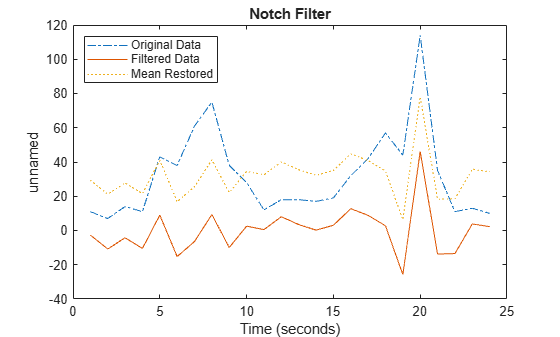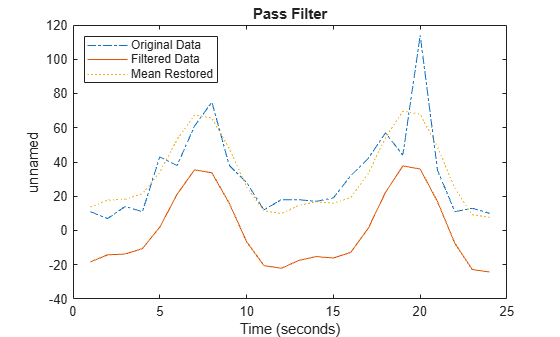# idealfilter

`timeseries` ideal filter

## Syntax

``tsout = idealfilter(tsin,interval,filtertype)``
``tsout = idealfilter(tsin,interval,filtertype,ind)``

## Description

example

````tsout = idealfilter(tsin,interval,filtertype)` applies an ideal (noncausal) filter of type `filtertype` to the frequency intervals specified by `interval` for a `timeseries` object `tsin`.Ideal filters are noncausal, and the ends of the filter amplitude are flat in the frequency domain. The data in `ts` must have zero mean.```
````tsout = idealfilter(tsin,interval,filtertype,ind)` optionally specifies the row or column indices of `tsin` to apply the filter to.```

## Examples

collapse all

First apply an ideal notch filter to a `timeseries` object, then apply a pass filter.

Load the data in the file `count.dat`, and create a `timeseries` object from the matrix `count`.

```load count.dat tsin = timeseries(count(:,1),1:24);```

Compute the mean of the data in `tsin`.

`tsinmean = mean(tsin);`

Define the frequency interval, in hertz, for filtering the data.

`interval = [0.08 0.2];`

Invoke an ideal notch filter.

`tsoutnotch = idealfilter(tsin,interval,'notch');`

Compare the original data and the filtered data.

```plot(tsin,'-.') hold on plot(tsoutnotch,'-')```

Restore the mean to the filtered data.

```tsoutnotchmean = tsoutnotch + tsinmean; plot(tsoutnotchmean,':') title('Notch Filter') legend('Original Data','Filtered Data','Mean Restored',... 'Location','NorthWest') hold off ```Repeat the filtering process using a `pass` filter.

```plot(tsin,'-.') hold on tsoutpass = idealfilter(tsin,interval,'pass'); plot(tsoutpass,'-') tsoutpassmean = tsoutpass + tsinmean; plot(tsoutpassmean,':') title('Pass Filter') legend('Original Data','Filtered Data','Mean Restored',... 'Location','NorthWest')```## Input Arguments

collapse all

Input `timeseries` with zero mean, specified as a scalar.

• If `tsin` is nonuniformly sampled, then `idealfilter` resamples the data on a uniform time vector before applying the filter.

• `idealfilter` replaces any `NaN` elements of `tsin` using the interpolation method associated with `tsin` prior to applying the filter.

Data Types: `timeseries`

Frequency interval, specified as a two-column matrix where each row represents the start and end frequencies for each interval.

Data Types: `double` | `single` | `int8` | `int16` | `int32` | `int64` | `uint8` | `uint16` | `uint32` | `uint64`

Filter type, specified as one of the following options:

• `'pass'` — Allow variations in a specific frequency range

• `'notch'` — Remove variations in a specific frequency range

Data Types: `double` | `single` | `int8` | `int16` | `int32` | `int64` | `uint8` | `uint16` | `uint32` | `uint64`

Row or column indices, specified as a positive integer numeric scalar or vector. `ind` represents column indices for column-oriented data (`tsin.IsTimeFirst` is `true`) and represents row indices for row-oriented data (`tsin.IsTimeFirst` is `false`).

Data Types: `double` | `single` | `int8` | `int16` | `int32` | `int64` | `uint8` | `uint16` | `uint32` | `uint64`